## Monday, August 22, 2011

### Metatron's Cube

Metatron is an angel recognized in both the Jewish and Christian religions as the Heavenly Scribe.
THE PLATONIC SOLIDS,
in geometry, are regular convex polyhedrons.
All of their faces, edges, vertices, and angles are congruent.
There are 5 of them.
They are named after Plato because he theorized that there are 5 elements,
all constructed out of regular solids.

Interestingly, in chemistry, it has been discovered that
every element in the periodic table can be fit molecularly
to fit one or all of the platonic solids.

Quote from Timaeus:

"The first will be the simplest and smallest construction, and its element is that triangle which has its hypotenuse twice the lesser side. When two such triangles are joined at the diagonal, and this is repeated three times, and the triangles rest their diagonals and shorter sides on the same point as a centre, a single equilateral triangle is formed out of six triangles ; and four equilateral triangles, if put together, make out of every three plane angles one solid angle, being that which is nearest to the most obtuse of plane angles ; and out of the combination of these four angles arises the first solid form which distributes into equal and similar parts the whole circle in which it is inscribed. The second species of solid is formed out of the same triangles, which unite as eight equilateral triangles and form one solid angle out of four plane angles, and out of six such angles the second body is completed. And the third body is made up of 120 triangular elements, forming twelve solid angles, each of them included in five plane equilateral triangles, having altogether twenty bases, each of which is an equilateral triangle. The one element [that is, the triangle which has its hypotenuse twice the lesser side] having generated these figures, generated no more ; but the isosceles triangle produced the fourth elementary figure, which is compounded of four such triangles, joining their right angles in a centre, and forming one equilateral quadrangle. Six of these united form eight solid angles, each of which is made by the combination of three plane right angles ; the figure of the body thus composed is a cube, having six plane quadrangular equilateral bases. There was yet a fifth combination which God used in the delineation of the universe."

"To earth, then, let us assign the cubical form ; for earth is the most immoveable of the four and the most plastic of all bodies, and that which has the most stable bases must of necessity be of such a nature. Now, of the triangles which we assumed at first, that which has two equal sides is by nature more firmly based than that which has unequal sides ; and of the compound figures which are formed out of either, the plane equilateral quadrangle has necessarily, a more stable basis than the equilateral triangle, both in the whole and in the parts. Wherefore, in assigning this figure to earth, we adhere to probability ; and to water we assign that one of the remaining forms which is the least moveable ; and the most moveable of them to fire ; and to air that which is intermediate. Also we assign the smallest body to fire, and the greatest to water, and the intermediate in size to air ; and, again, the acutest body to fire, and the next in acuteness to, air, and the third to water. Of all these elements, that which has the fewest bases must necessarily be the most moveable, for it must be the acutest and most penetrating in every way, and also the lightest as being composed of the smallest number of similar particles : and the second body has similar properties in a second degree, and the third body in the third degree. Let it be agreed, then, both according to strict reason and according to probability, that the pyramid is the solid which is the original element and seed of fire ; and let us assign the element which was next in the order of generation to air, and the third to water. We must imagine all these to be so small that no single particle of any of the four kinds is seen by us on account of their smallness : but when many of them are collected together their aggregates are seen. And the ratios of their numbers, motions, and other properties, everywhere God, as far as necessity allowed or gave consent, has exactly perfected, and harmonised in due proportion."

THE PLATONIC SOLIDS

1) Tetrahedron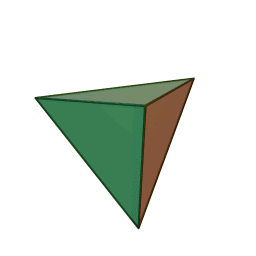1 dimension 4 sides 4 vertices Element: FIRE

In Metatron's Cube

Star Tetrahedron (Merkabah)

2) Hexahedron (Cube)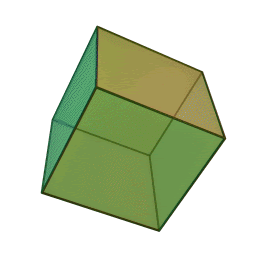2 dimensions 6 sides 8 vertices Element: EARTH
In Metatron's Cube

3) Octahedron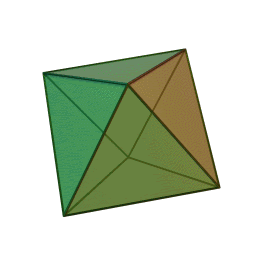3 dimensions 8 sides 6 vertices Element: AIR
In Metatron's Cube

4) Icosahedron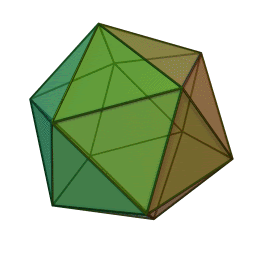4 dimensions 20 sides 12 vertices Element: WATER
In Metatron's Cube

5) Dodecahedron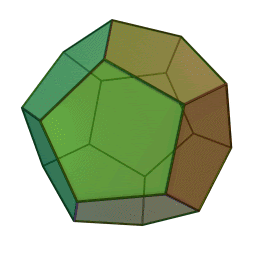5 dimensions 12 sides 20 vertices Element: ETHER/UNIVERSE

In Metatron's Cube

These elements (earth, fire, air, water, and universe/void/ether) make up our observable physical reality.

#### 1 comment:

1.It's deceptive and incorrect to say that the hexahedron is "2 dimensions" and the octahedron is "3 dimensions" and so forth. All of these objects are two dimensional (because they are on a 2D screen). They are 2D projections of three dimensional objects. All have a symmetry class. The dodecahedron and the icosahedron share icosahedral (5-fold) symmetry. The octahedron and hexahedron share octahedral (4-fold) symmetry. The tetrahedron has tetrahedral symmetry.